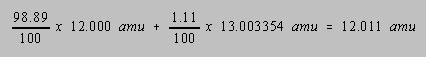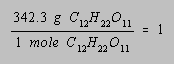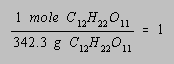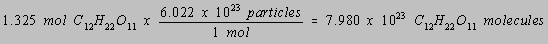## Definition of Terms

 Atomic Mass Isotopes Atomic Weight The Mole Molecular Weight Avogadro's Constant Converting Grams Into Moles

Atomic Mass

The units in which the mass of an atom are expressed are atomic mass units. At one time, the lightest atom was assigned a mass of 1 amu and the mass of any other atom was expressed in terms of this standard. Today atomic mass units are defined in terms of the 12C isotope, which is assigned a mass of exactly 12.000... amu.Isotopes

Isotopes are atoms of the same element with different numbers of neutrons, such as the 20Ne and 22Ne isotopes of neon or the 35Cl and 37Cl isotopes of chlorine.Atomic Weight

The atomic weight of an element is the weighted average of the atomic masses of the different isotopes of an element. Naturally occurring carbon, for example, is a mixture of two isotopes, 12C (98.89%) and 13C (1.11 %). Individual carbon atoms therefore have a mass of either 12.000 or 13.03354 amu. But the average mass of the different isotopes of carbon is 12.011 amu.Molecular Weight

The molecular weight of a compound is the sum of the atomic weights of the atoms in the molecules that form these compounds.

Example: The molecular weight of the sugar molecule found in cane sugar is the sum of the atomic weights of the 12 carbon atoms, 22 hydrogen atoms, and 11 oxygen atoms in a C12H22O11 molecule.

 12 C atoms = 12(12.011) amu = 144.132 amu 22 H atoms = 22(1.0079) amu = 22.174 amu 11 O atoms = 11(15.9994) amu = 175.993 amu 342.299 amu

C12H22O11 has a molecular weight of 342.299 amu. A mole of C12H22O11 would have a mass of 342.299 grams.This quantity is known as the molar mass, a term that is often used in place of the terms atomic weight or molecular weight.The Mole

The term mole literally means a small mass. It is used as the bridge between chemistry on the atomic and macroscopic scale. If the mass of a single 12C atom is 12.000 amu, then one mole of these atoms would have a mass of 12.000 grams. By definition, aa mole of any substance contains the same number of elementary particles as there are atoms in exactly 12 grams of the 12C isotope of carbon.

Example: A single 12C atom has a mass of 12 amu, and a mole of these atoms would have a mass of 12 grams.

A mole of any atoms has a mass in grams equal to the atomic weight of the element. The term mole can be applied to any particle: atoms, a mole of atoms, a mole of ions, a mole of electrons, or a mole of molecules. Each time we use the term, we refer to a number of particles equal to the number of atoms in exactly 12 grams of the 12C isotope of carbon.

 Practice Problem 1:Predict the mass of a mole of magnesium atoms. Click here to check your anwswer to Practice Problem 1

 Practice Problem 2:Calculate the molecular weights of carbon dioxide (CO2) and sugar (C12H22O11) and the mass of a mole of each compound. Click here to check your answer to Practice Problem 2 Click here to see a solution to Practice Problem 2Avogadro's Constant

Avogadro's number (or Avogadro's constant) is the number of elementary particles in a mole of any substance For most calculations, four significant figures for Avogadro's constant are enough: 6.022 x 1023.

A mole of any substance contains Avogadro's number of elementary particles. It doesn't matter whether we talk about a mole of atoms, a mole of molecules, a mole of electrons, or a mole of ions. By definition, a mole always contains 6.022 x 1023 elementary particles.

 Practice Problem 3:Describe the difference between the mass of a mole of oxygen atoms and a mole of O2 molecules. Click here to check your answer to Practice Problem 3

 Practice Problem 4:Calculate the mass in grams of a single 12C atom. Click here to check your answer to Practice Problem 4 Click here to see a solution to Practice Problem 4Converting Grams into Moles

The mole is a powerful tool, which enables chemists armed with nothing more than a table of atomic weights and a balance to determine the number of atoms, ions or molecules in a sample.

Example: Let's calculate the number of C12H22O11 molecules in a pound of cane sugar.

We need two pieces of information to do this calculation. We need to know the number of grams in a pound and the molecular weight or molar mass of this sugar. A pound of sugar has a mass of 453.6 grams and one mole of this sugar has a mass of 342.3 grams.

A pair of unit factors can be constructed from the molecular weight or molar mass.By paying attention to the units during the calculation, it is easy to choose the correct unit factor to convert grams of sugar into moles of sugar.We then use Avogadro's number to determine the number of C12H22O11 molecules in our sample.Practice Problem 5:What is the formula of magnesium chloride if 2.55 grams of magnesium combines with 7.45 grams of chlorine to form 10.0 grams of this compound? Click here to check your answer to Practice Problem 5 Click here to see a solution to Practice Problem 5